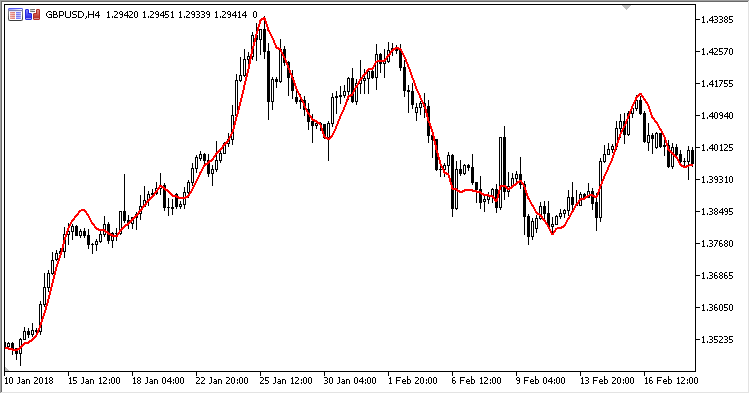320

2018.10.02 08:55

• Period - 计算周期
• Applied price

```MMM = MA + 6 * Slope / K
```

```MA - SMA(Applied price, Period)
Slope = Period  范围内 (Applied price * (Period-Factor)/2) 的累计
Factor = 1 + 2*(N-1)
N = 'Period' 范围内从 1 到 Period 的值
K = Period*(Period+1)
```MA_Signal

MA 信号指标Investor_Preference_IndexNRMA

Nick Rypoсk 移动平均线指标T3

T3 移动平均线指标